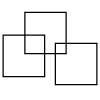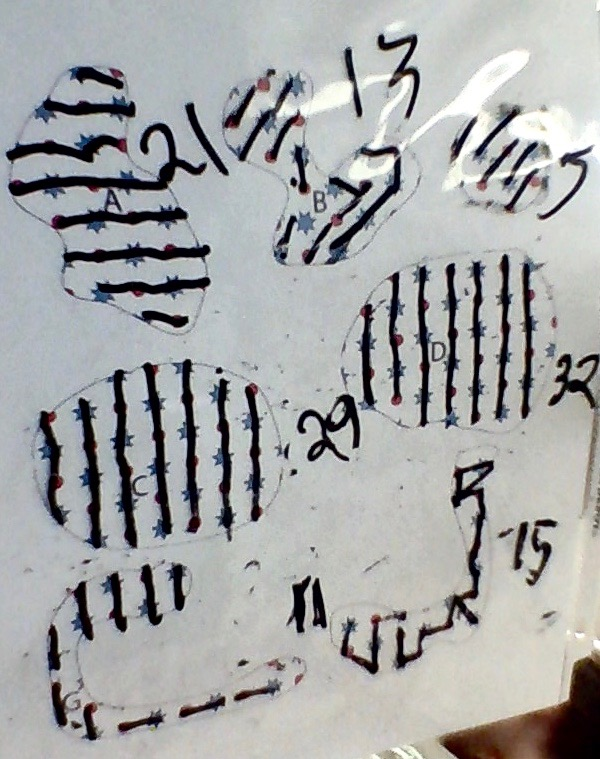#### You may also like### Three Squares

What is the greatest number of squares you can make by overlapping three squares?### Two Dice

Find all the numbers that can be made by adding the dots on two dice.### Biscuit Decorations

Andrew decorated 20 biscuits to take to a party. He lined them up and put icing on every second biscuit and different decorations on other biscuits. How many biscuits weren't decorated?

# Wallpaper

##### Age 5 to 7Challenge Level

Ayati from Chippenham Primary School wrote:

From smallest to largest, I have worked out the size order as E, G, B, F, A, C, D. I did this by counting how many complete blue stars there were in each scrap of wallpaper. It was easier and more efficient.

Jonah and Adeline from Pratt Community School in the United States sent in this excellent explanation:

First, we boxed each shape and we measured the perimeter of all of the shapes. We measured in inches. But this did not work, because the shapes are curved and it is difficult to box the shapes precisely. As a consequence, we could not find a precise perimeter. For example, it is easier to box D, E and C - because these are oval or round shapes. Shapes like G and F were difficult to find the accurate measurement. We thought about - what if we could stretch G and F out, to an easier shape to find the perimeter. But then we realized that the shapes would have the same number of dots, whether they were stretched out or not.

So, we tried something different. We thought that the stars and dots would help us find the area of each shape. First, we counted all the whole stars and whole dots. Then we realized that we could add the half shapes together to make another whole.

Based on counting the dots and stars, here is the order from smallest to biggest:

E = 10    G = 19     B & F (tied) = 20     A = 24     C = 33     D = 39

Bea, from the same school, sent in the following together with a picture:

First, I tried to imagine that I could fold the shapes so that they would be similar. For example, I imagined that I could fold the top of F down so that it would be similar to C and D.
I realized that no matter how much I stretched it, it would not give me an accurate measure of the perimeter. So, I decided to look at the stars and the dots - the inside. I drew lines from shape to shape. A line from star to dot and dot to star counted as 1 line segment. I followed that strategy with every shape.
Then I counted the line segments. Here's the order from smallest to biggest:

E = 5     G = 11     B = 13     F = 15     A = 21     C = 29     D = 32Thank you for all the solutions.  B and F are very similar in size, so we think they could go either way round in the order.